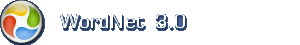# English dictionary

Hint: In most browsers you can lookup any word by double click it.

## English noun: ratio

1. ratio the relative magnitudes of two quantities (usually expressed as a quotient)

Narrower (hyponym)abundance, abundance, albedo, aspect ratio, average, betting odds, breadth index, cephalic index, cranial index, eccentricity, efficiency, employee turnover, f number, facial index, factor of safety, focal ratio, frequency, fuel consumption rate, gas mileage, gasoline mileage, gross margin, haematocrit, hematocrit, I.Q., index of refraction, intelligence quotient, IQ, load factor, loading, loss ratio, Mach number, magnification, magnitude, margin of profit, mechanical advantage, mileage, odds, order of magnitude, output-to-input ratio, P/E ratio, packed cell volume, prevalence, price-to-earnings ratio, productivity, profit margin, proportionality, quotient, reflective power, refractive index, relative frequency, relative humidity, respiratory quotient, S/N, safety factor, signal-to-noise, signal-to-noise ratio, signal/noise, signal/noise ratio, speed, stoichiometry, stop number, time constant, turnover, turnover rate

2. ratio the relation between things (or parts of things) with respect to their comparative quantity, magnitude, or degree

SamplesAn inordinate proportion of the book is given over to quotations.
A dry martini has a large proportion of gin.

Synonymsproportion